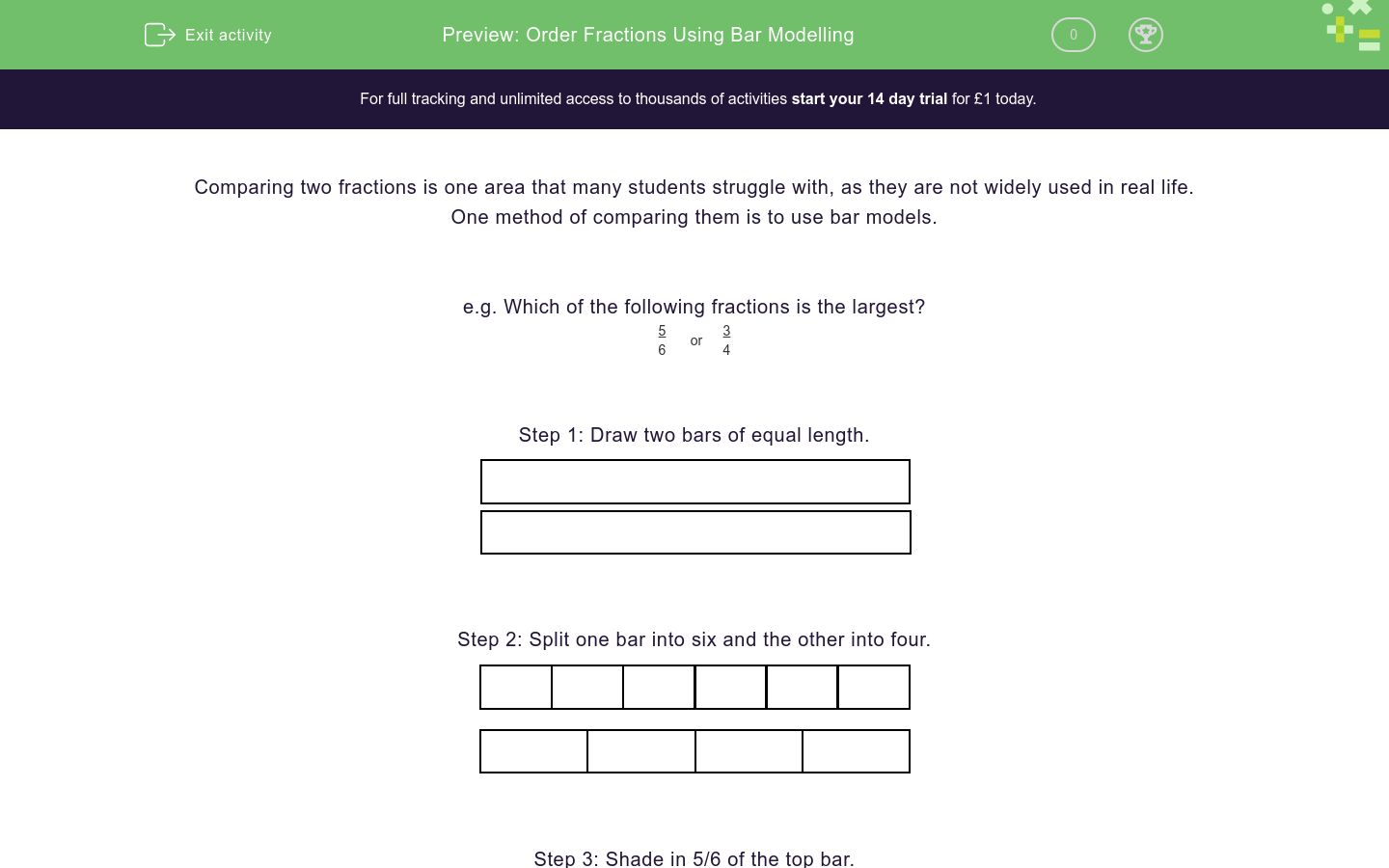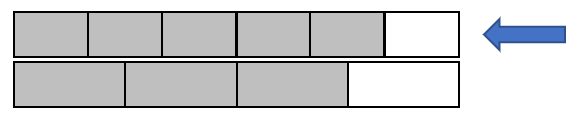# Order Fractions Using Bar Modelling

In this worksheet, students will use bar models to compare and order fractions in terms of magnitude.Key stage:  KS 4

GCSE Subjects:   Maths

GCSE Boards:   Pearson Edexcel, OCR, Eduqas, AQA

Curriculum topic:   Number, Fractions, Decimals and Percentages

Curriculum subtopic:   Structure and Calculation, Ordering Fractions, Decimals and Percentages

Difficulty level:### QUESTION 1 of 10

Comparing two fractions is one area that many students struggle with, as they are not widely used in real life.

One method of comparing them is to use bar models.

e.g. Which of the following fractions is the largest?

 5 6
or
 3 4

Step 1: Draw two bars of equal length.Step 2: Split one bar into six and the other into four.Step 3: Shade in 5/6 of the top bar.Step 4: Shade in 3/4 of the lower bar.Step 5: Compare the fractions to decide which one is bigger.As you can see, 5/6 is larger, as more of its bar has been coloured and the bars are exactly the same length.

In this activity, you will compare pairs of fractions in order to choose which is larger.

You will want to have some paper and a pencil with you so that you can create your own bar models as we work through these challenges together.

Which of the fractions below is the largest?

 1 2
or
 1 3
1/2

1/3

Which of the fractions below is the largest?

 3 5
or
 2 3
3/5

2/3

Which of the fractions below is the largest?

 4 5
or
 5 6
4/5

5/6

Which of the fractions below is the largest?

 9 10
or
 4 5
9/10

4/5

Which of the fractions below is the largest?

 3 7
or
 2 5
3/7

2/5

Which of the fractions below is the largest?

 5 8
or
 3 5
5/8

3/5

Which of the fractions below is the largest?

 4 9
or
 3 8
4/9

3/8

Which of the fractions below is the largest?

 6 10
or
 2 3
6/10

2/3

Which of the fractions below is the largest?

 3 7
or
 4 9
3/7

4/9

Which of the fractions below is the largest?

 2 5
or
 1 3
2/5

1/3

• Question 1

Which of the fractions below is the largest?

 1 2
or
 1 3
1/2
EDDIE SAYS
Did your drawings look something like this?As we can see here, 1/2 is visibly larger than 1/3.
• Question 2

Which of the fractions below is the largest?

 3 5
or
 2 3
2/3
EDDIE SAYS
You can see the bar below, which represents 2/3 stretches further than 3/5.Is this becoming less daunting?
• Question 3

Which of the fractions below is the largest?

 4 5
or
 5 6
5/6
EDDIE SAYSEven though these areas are much closer to each other, the 5/6 bar is very slightly longer. Did you spot that?
• Question 4

Which of the fractions below is the largest?

 9 10
or
 4 5
9/10
EDDIE SAYS
Your drawings should look something like this:The 9/10 bar extends further than 4/5, indicating that this is larger.
• Question 5

Which of the fractions below is the largest?

 3 7
or
 2 5
3/7
EDDIE SAYS
Another close one here:However, the 3/7 bar is slightly longer so this is our larger fraction. This is good work so far, keep it up!
• Question 6

Which of the fractions below is the largest?

 5 8
or
 3 5
5/8
EDDIE SAYS
Your bars should resemble the two below:Can you spot which is larger here?
• Question 7

Which of the fractions below is the largest?

 4 9
or
 3 8
4/9
EDDIE SAYSGreat focus!
• Question 8

Which of the fractions below is the largest?

 6 10
or
 2 3
2/3
EDDIE SAYSKeep practising, are you starting to feel more confident?
• Question 9

Which of the fractions below is the largest?

 3 7
or
 4 9
4/9
EDDIE SAYS• Question 10

Which of the fractions below is the largest?

 2 5
or
 1 3
2/5
EDDIE SAYSGreat work, you've completed another activity and mastered ordering fractions using the bar method! Why not attempt the next exercise, as you're on a roll?
---- OR ----

Sign up for a £1 trial so you can track and measure your child's progress on this activity.

### What is EdPlace?

We're your National Curriculum aligned online education content provider helping each child succeed in English, maths and science from year 1 to GCSE. With an EdPlace account you’ll be able to track and measure progress, helping each child achieve their best. We build confidence and attainment by personalising each child’s learning at a level that suits them.

Get started